"道德是用来束缚自己的，不是用来绑架别人的 "

#  AMLP-Models# Overfitting and Underfitting

Overfitting: can not generalize well

Underfitting

# Feature Normalization

Why: Make sure that all features are in same scale.

MinMaxScaler: scale all feature and transform them to 0-1

sklearn.preprocessing.MinMaxScaler — scikit-learn 0.24.2 documentation

scaler.fit_transform(X_train)

scaler.transform(X_train)

from sklearn.preprocessing import MinMaxScaler
scaler = MinMaxScaler()
X_train_scaled = scaler.fit_transform(X_train)

# we must apply the scaling to the test set that we computed for the training set
X_test_scaled = scaler.transform(X_test)

#knn = KNeighborsClassifier(n_neighbors = 5)
#knn.fit(X_train_scaled, y_train)
#print('Accuracy of K-NN classifier on training set: {:.2f}'
#     .format(knn.score(X_train_scaled, y_train)))
#print('Accuracy of K-NN classifier on test set: {:.2f}'
#     .format(knn.score(X_test_scaled, y_test)))

#example_fruit = [[5.5, 2.2, 10, 0.70]]
#example_fruit_scaled = scaler.transform(example_fruit)
#print('Predicted fruit type for ', example_fruit, ' is ',
#target_names_fruits[knn.predict(example_fruit_scaled)-1])



Tip: the test set must use identical scaling to the training set

# Cross Validation

Details of Cross Validation will be released later.

sklearn.model_selection.cross_val_score — scikit-learn 0.24.2 documentation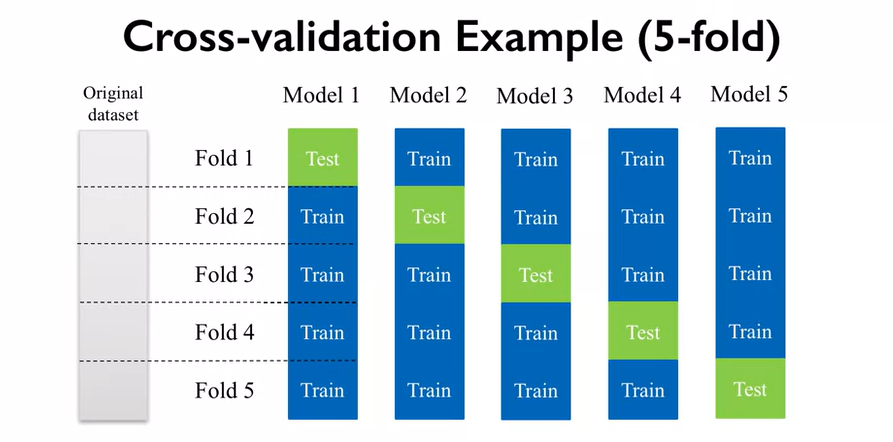• Stratified Cross-validation: Each fold contains a proportion of classes that matches the overall dataset.
• Leave-one-out cross-validation: Each fold consists of a single sample as the test set.(better for small sets)

Example based on k-NN classifier with fruit dataset (2 features)

from sklearn.model_selection import cross_val_score

clf = KNeighborsClassifier(n_neighbors = 5)
X = X_fruits_2d.as_matrix()
y = y_fruits_2d.as_matrix()
cv_scores = cross_val_score(clf, X, y)

print('Cross-validation scores (3-fold):', cv_scores)
print('Mean cross-validation score (3-fold): {:.3f}'
.format(np.mean(cv_scores)))


Cross-validation scores (3-fold): [ 0.77  0.74  0.83]
Mean cross-validation score (3-fold): 0.781


# Validation Curve

Details of Cross Validation will be released later.

sklearn.model_selection.validation_curve — scikit-learn 0.24.2 documentation

from sklearn.svm import SVC
from sklearn.model_selection import validation_curve

param_range = np.logspace(-3, 3, 4)
train_scores, test_scores = validation_curve(SVC(), X, y,
param_name='gamma',
param_range=param_range, cv=3)
print(train_scores)
print(test_scores)
# This code based on scikit-learn validation_plot example
#  See:  http://scikit-learn.org/stable/auto_examples/model_selection/plot_validation_curve.html
plt.figure()

train_scores_mean = np.mean(train_scores, axis=1)
train_scores_std = np.std(train_scores, axis=1)
test_scores_mean = np.mean(test_scores, axis=1)
test_scores_std = np.std(test_scores, axis=1)

plt.title('Validation Curve with SVM')
plt.xlabel('$\gamma$ (gamma)')
plt.ylabel('Score')
plt.ylim(0.0, 1.1)
lw = 2

plt.semilogx(param_range, train_scores_mean, label='Training score',
color='darkorange', lw=lw)

plt.fill_between(param_range, train_scores_mean - train_scores_std,
train_scores_mean + train_scores_std, alpha=0.2,
color='darkorange', lw=lw)

plt.semilogx(param_range, test_scores_mean, label='Cross-validation score',
color='navy', lw=lw)

plt.fill_between(param_range, test_scores_mean - test_scores_std,
test_scores_mean + test_scores_std, alpha=0.2,
color='navy', lw=lw)

plt.legend(loc='best')
plt.show()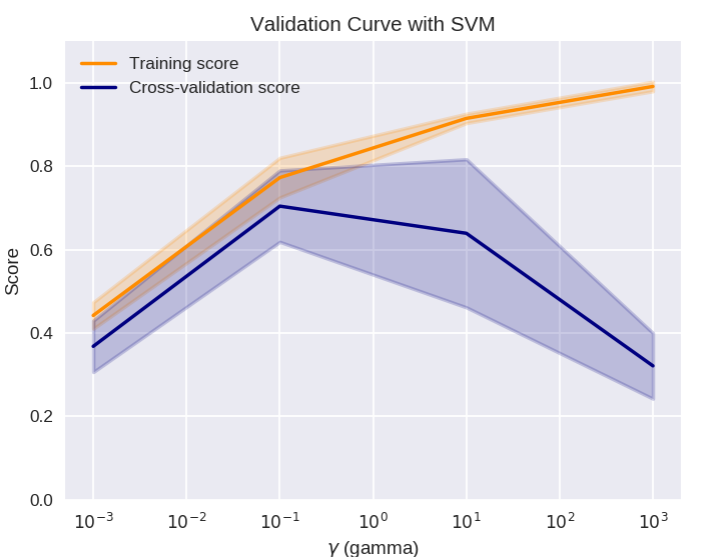# Feature importance

sklearn.ensemble.RandomForestRegressor — scikit-learn 0.24.2 documentation

## Details of feature importance

How important is a feature to overall prediction accuracy

There are indeed several ways to get feature “importances”. As often, there is no strict consensus about what this word means.
In scikit-learn, we implement the importance as described in 1 (often cited, but unfortunately rarely read…). It is sometimes called “gini importance” or “mean decrease impurity” and is defined as the total decrease in node impurity (weighted by the probability of reaching that node (which is approximated by the proportion of samples reaching that node)) averaged over all trees of the ensemble.
In the literature or in some other packages, you can also find feature importances implemented as the “mean decrease accuracy”. Basically, the idea is to measure the decrease in accuracy on OOB data when you randomly permute the values for that feature. If the decrease is low, then the feature is not important, and vice-versa.
(Note that both algorithms are available in the randomForest R package.)

Two ways to evaluate feature importance2

1. Mean decrease impurity
2. Mean decrease accuracy3

## Application

from adspy_shared_utilities import plot_feature_importances

plt.figure(figsize=(10,4), dpi=80)
plot_feature_importances(clf, iris.feature_names)
plt.show()

print('Feature importances: {}'.format(clf.feature_importances_))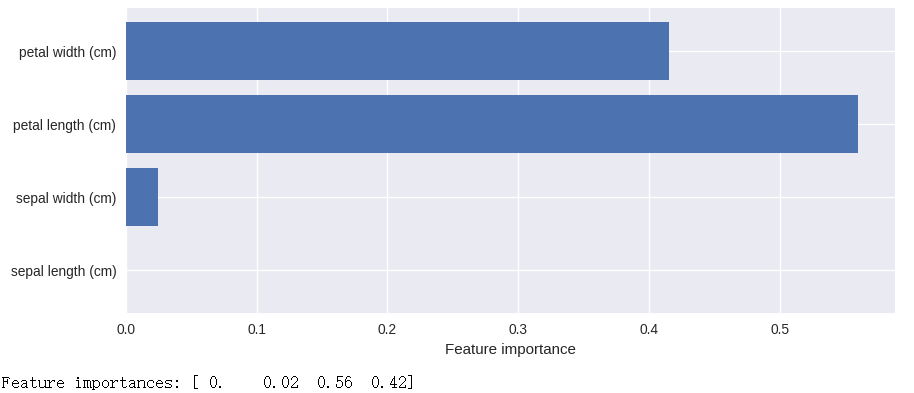# K-Nearest Neighbors(KNN) Model

## Classification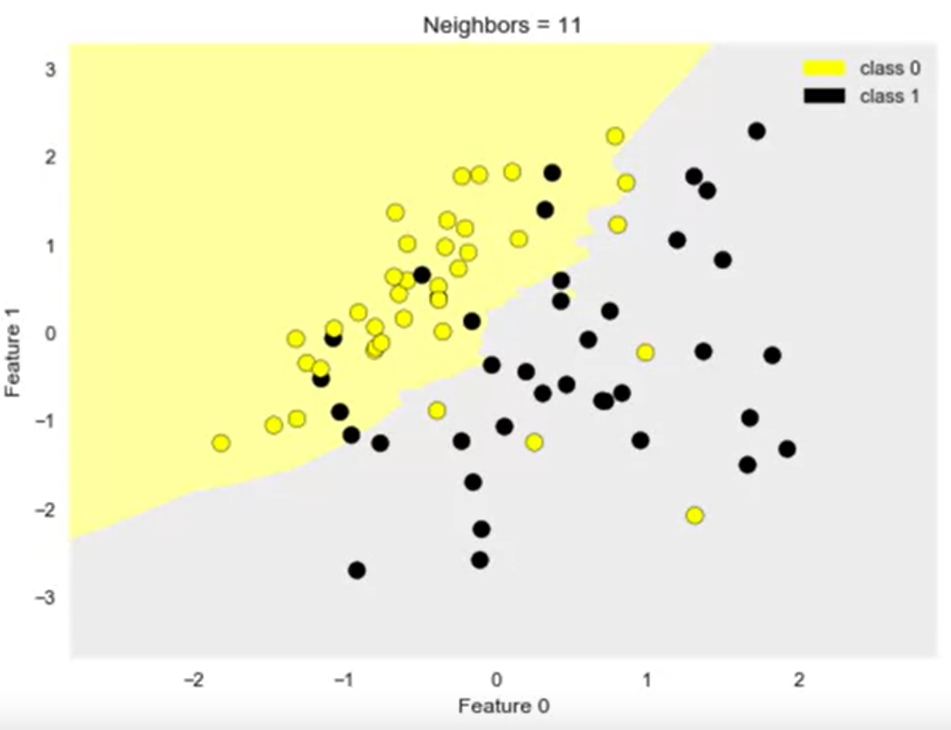from adspy_shared_utilities import plot_two_class_knn

X_train, X_test, y_train, y_test = train_test_split(X_C2, y_C2,
random_state=0)

plot_two_class_knn(X_train, y_train, 1, 'uniform', X_test, y_test)
plot_two_class_knn(X_train, y_train, 3, 'uniform', X_test, y_test)
plot_two_class_knn(X_train, y_train, 11, 'uniform', X_test, y_test)


## Regression

sklearn.neighbors.KNeighborsRegressor — scikit-learn 0.24.2 documentation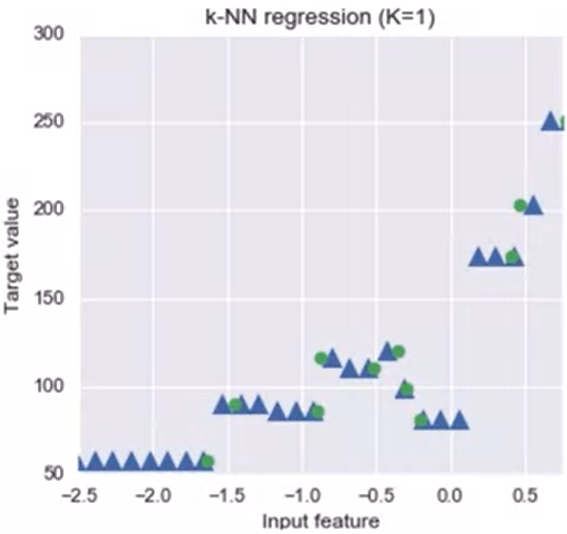🔴 : training values

🔺 : predicted values

from sklearn.neighbors import KNeighborsRegressor

X_train, X_test, y_train, y_test = train_test_split(X_R1, y_R1, random_state = 0)

knnreg = KNeighborsRegressor(n_neighbors = 5).fit(X_train, y_train)

#print(knnreg.predict(X_test))
print('R-squared test score: {:.3f}'
.format(knnreg.score(X_test, y_test)))

fig, subaxes = plt.subplots(1, 2, figsize=(8,4))
X_predict_input = np.linspace(-3, 3, 50).reshape(-1,1)
X_train, X_test, y_train, y_test = train_test_split(X_R1[0::5], y_R1[0::5], random_state = 0)

for thisaxis, K in zip(subaxes, [1, 3]):
knnreg = KNeighborsRegressor(n_neighbors = K).fit(X_train, y_train)
y_predict_output = knnreg.predict(X_predict_input)
thisaxis.set_xlim([-2.5, 0.75])
thisaxis.plot(X_predict_input, y_predict_output, '^', markersize = 10,
label='Predicted', alpha=0.8)
thisaxis.plot(X_train, y_train, 'o', label='True Value', alpha=0.8)
thisaxis.set_xlabel('Input feature')
thisaxis.set_ylabel('Target value')
thisaxis.set_title('KNN regression (K={})'.format(K))
thisaxis.legend()
plt.tight_layout()


## Evaluation

r-squared regression score: Measure how well a prediction model for regression fits the given data

• range: 0~1
• 0: a constant model that predicts the mean value of al training target values
• 1: perfect prediction

coefficient of determination

Regression model complexity as a function of K

# plot k-NN regression on sample dataset for different values of K
fig, subaxes = plt.subplots(5, 1, figsize=(5,20))
X_predict_input = np.linspace(-3, 3, 500).reshape(-1,1)
X_train, X_test, y_train, y_test = train_test_split(X_R1, y_R1,
random_state = 0)

for thisaxis, K in zip(subaxes, [1, 3, 7, 15, 55]):
knnreg = KNeighborsRegressor(n_neighbors = K).fit(X_train, y_train)
y_predict_output = knnreg.predict(X_predict_input)
train_score = knnreg.score(X_train, y_train)
test_score = knnreg.score(X_test, y_test)
thisaxis.plot(X_predict_input, y_predict_output)
thisaxis.plot(X_train, y_train, 'o', alpha=0.9, label='Train')
thisaxis.plot(X_test, y_test, '^', alpha=0.9, label='Test')
thisaxis.set_xlabel('Input feature')
thisaxis.set_ylabel('Target value')
thisaxis.set_title('KNN Regression (K={})\n\
Train $R^2 = {:.3f}$,  Test $R^2 = {:.3f}$'
.format(K, train_score, test_score))
thisaxis.legend()


# Linear Models

A linear model is a sum of weighted variables that predicts a target output value given an input data instance

Linear Regression is an example:

Details of Linear Models（Linear Regression)

## Least-squares linear regression(ordinary least-squares)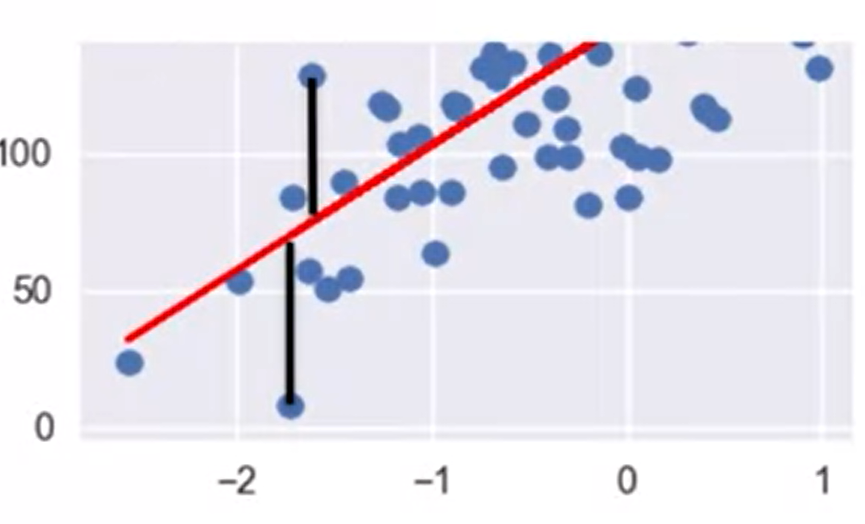objective function

loss function

## Ridge regression

sklearn.linear_model.Ridge — scikit-learn 0.24.2 documentation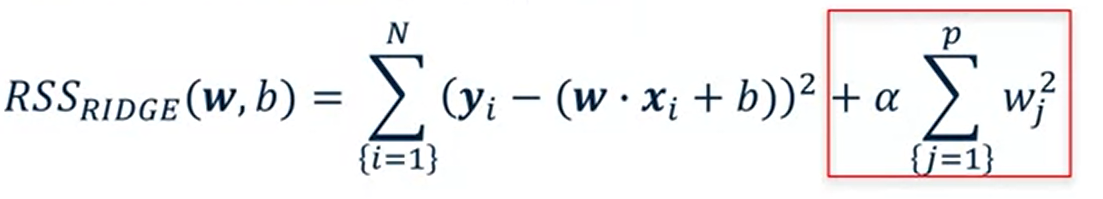The addition of a parameter penalty (also called regularization): reduce complexity

Uses L2 regularization: minimize sum of squares of w entries

from sklearn.linear_model import Ridge
X_train, X_test, y_train, y_test = train_test_split(X_crime, y_crime,
random_state = 0)

linridge = Ridge(alpha=20.0).fit(X_train, y_train)

#print('Crime dataset')
#print('ridge regression linear model intercept: {}'
#     .format(linridge.intercept_))
#print('ridge regression linear model coeff:\n{}'
#     .format(linridge.coef_))
#print('R-squared score (training): {:.3f}'
#     .format(linridge.score(X_train, y_train)))
#print('R-squared score (test): {:.3f}'
#     .format(linridge.score(X_test, y_test)))
#print('Number of non-zero features: {}'
#     .format(np.sum(linridge.coef_ != 0)))



## Lasso regression

sklearn.linear_model.Lasso — scikit-learn 0.24.2 documentation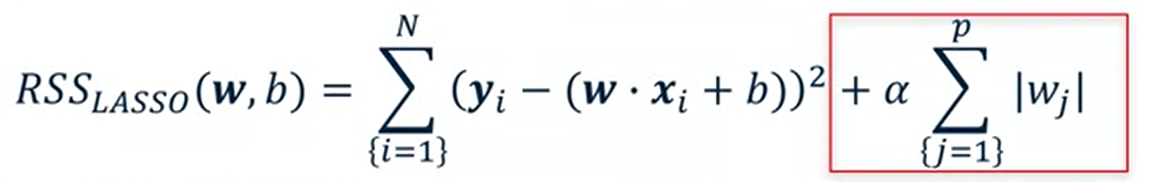Uses L1 regularization

from sklearn.linear_model import Lasso
from sklearn.preprocessing import MinMaxScaler
scaler = MinMaxScaler()

X_train, X_test, y_train, y_test = train_test_split(X_crime, y_crime,
random_state = 0)

X_train_scaled = scaler.fit_transform(X_train)
X_test_scaled = scaler.transform(X_test)

linlasso = Lasso(alpha=2.0, max_iter = 10000).fit(X_train_scaled, y_train)

#print('Crime dataset')
#print('lasso regression linear model intercept: {}'
#     .format(linlasso.intercept_))
#print('lasso regression linear model coeff:\n{}'
#     .format(linlasso.coef_))
#print('Non-zero features: {}'
#     .format(np.sum(linlasso.coef_ != 0)))
#print('R-squared score (training): {:.3f}'
#     .format(linlasso.score(X_train_scaled, y_train)))
#print('R-squared score (test): {:.3f}\n'
#     .format(linlasso.score(X_test_scaled, y_test)))
#print('Features with non-zero weight (sorted by absolute magnitude):')

for e in sorted (list(zip(list(X_crime), linlasso.coef_)),
key = lambda e: -abs(e)):
if e != 0:
print('\t{}, {:.3f}'.format(e, e))


## Polynomial Regression

sklearn.preprocessing.PolynomialFeatures — scikit-learn 0.24.2 documentation

from sklearn.linear_model import LinearRegression
from sklearn.linear_model import Ridge
from sklearn.preprocessing import PolynomialFeatures

X_train, X_test, y_train, y_test = train_test_split(X_F1, y_F1,
random_state = 0)
linreg = LinearRegression().fit(X_train, y_train)

#Now we transform the original input data to add polynomial features up to degree 2 (quadratic)
poly = PolynomialFeatures(degree=2)

X_F1_poly = poly.fit_transform(X_F1)

X_train, X_test, y_train, y_test = train_test_split(X_F1_poly, y_F1,
random_state = 0)
linreg = LinearRegression().fit(X_train, y_train)

#Addition of many polynomial features often leads to overfitting, so we often use polynomial features in combination with regression that has a regularization penalty, like ridge regression.

X_train, X_test, y_train, y_test = train_test_split(X_F1_poly, y_F1,
random_state = 0)
linreg = Ridge().fit(X_train, y_train)



# Logistic regression

Details of Logistic Regression

sklearn.linear_model.LogisticRegression — scikit-learn 0.24.2 documentation

Example: Logistic regression for binary classification on fruits dataset using height, width features (positive class: apple, negative class: others)

from sklearn.linear_model import LogisticRegression
plot_class_regions_for_classifier_subplot)

fig, subaxes = plt.subplots(1, 1, figsize=(7, 5))
y_fruits_apple = y_fruits_2d == 1   # make into a binary problem: apples vs everything else
X_train, X_test, y_train, y_test = (
train_test_split(X_fruits_2d.as_matrix(),
y_fruits_apple.as_matrix(),
random_state = 0))

clf = LogisticRegression(C=100).fit(X_train, y_train)

plot_class_regions_for_classifier_subplot(clf, X_train, y_train, None,
None, 'Logistic regression \
for binary classification\nFruit dataset: Apple vs others',
subaxes)

h = 6
w = 8
print('A fruit with height {} and width {} is predicted to be: {}'
.format(h,w, ['not an apple', 'an apple'][clf.predict([[h,w]])]))

h = 10
w = 7
print('A fruit with height {} and width {} is predicted to be: {}'
.format(h,w, ['not an apple', 'an apple'][clf.predict([[h,w]])]))
subaxes.set_xlabel('height')
subaxes.set_ylabel('width')

print('Accuracy of Logistic regression classifier on training set: {:.2f}'
.format(clf.score(X_train, y_train)))
print('Accuracy of Logistic regression classifier on test set: {:.2f}'
.format(clf.score(X_test, y_test)))


: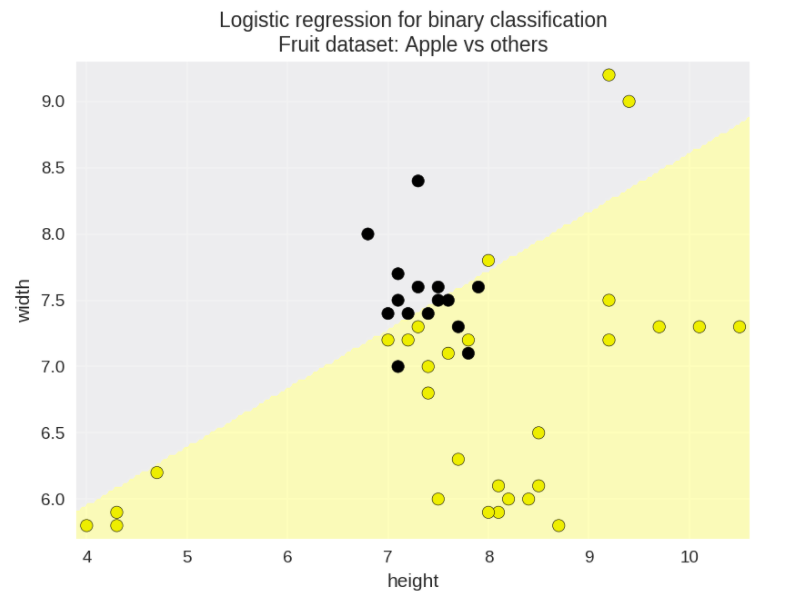• L2 is for regulariazaiton (default ‘on’)
• C controls amount of regularization(default 1.0)
• Normalization is very important as with regularized linear regression.

# Support Vector Machine

Details of svm will be released later.

## Linear Support Vector Machine

### Application

• f(x,w,b) = sign(w·x+b)
• Classifier margin:Defined as the maximum width the decision boundary area can be increased before hitting a data point
• Maximum Margin Linear Classifier : Linear Support Vector Machines(LSVM)
• C parameter: Regularization for SVMs
from sklearn.svm import SVC

X_train, X_test, y_train, y_test = train_test_split(X_C2, y_C2, random_state = 0)

fig, subaxes = plt.subplots(1, 1, figsize=(7, 5))
this_C = 1.0
clf = SVC(kernel = 'linear', C=this_C).fit(X_train, y_train)
title = 'Linear SVC, C = {:.3f}'.format(this_C)
plot_class_regions_for_classifier_subplot(clf, X_train, y_train, None, None, title, subaxes)


### Multi-class Classification(LSVM)

sklearn.svm.LinearSVC — scikit-learn 0.24.2 documentation

1. Classify M classes generates M one vs rest classifiers.
from sklearn.svm import LinearSVC

X_train, X_test, y_train, y_test = train_test_split(X_fruits_2d, y_fruits_2d, random_state = 0)

clf = LinearSVC(C=5, random_state = 67).fit(X_train, y_train)
print('Coefficients:\n', clf.coef_)
print('Intercepts:\n', clf.intercept_)


:
Coefficients:
[[-0.26 0.71]
[-1.63 1.16]
[ 0.03 0.29]
[ 1.24 -1.64]]
Intercepts:
[-3.29 1.2 -2.72 1.16]

1. iterate
plt.figure(figsize=(6,6))
colors = ['r', 'g', 'b', 'y']
cmap_fruits = ListedColormap(['#FF0000', '#00FF00', '#0000FF','#FFFF00'])

plt.scatter(X_fruits_2d[['height']], X_fruits_2d[['width']],
c=y_fruits_2d, cmap=cmap_fruits, edgecolor = 'black', alpha=.7)

x_0_range = np.linspace(-10, 15)

for w, b, color in zip(clf.coef_, clf.intercept_, ['r', 'g', 'b', 'y']):
# Since class prediction with a linear model uses the formula y = w_0 x_0 + w_1 x_1 + b,
# and the decision boundary is defined as being all points with y = 0, to plot x_1 as a
# function of x_0 we just solve w_0 x_0 + w_1 x_1 + b = 0 for x_1:
plt.plot(x_0_range, -(x_0_range * w + b) / w, c=color, alpha=.8)

plt.legend(target_names_fruits)
plt.xlabel('height')
plt.ylabel('width')
plt.xlim(-2, 12)
plt.ylim(-2, 15)
plt.show()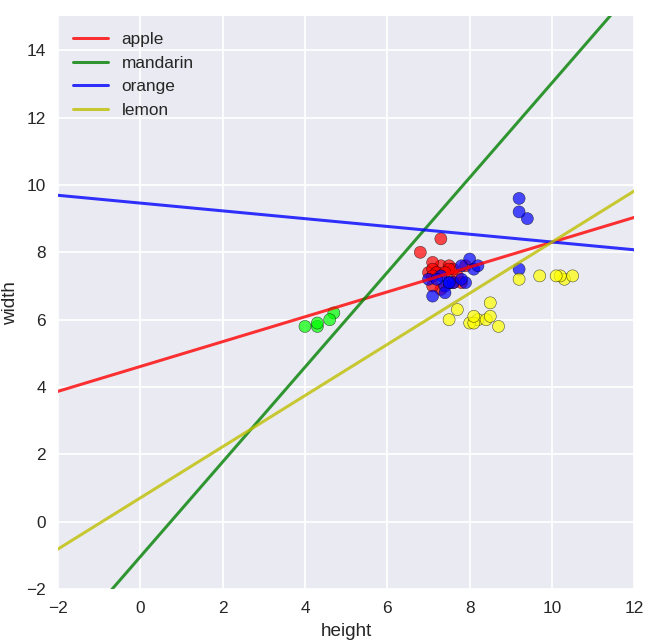### Pros and Cons

LSVM maynot solve classification problems like this: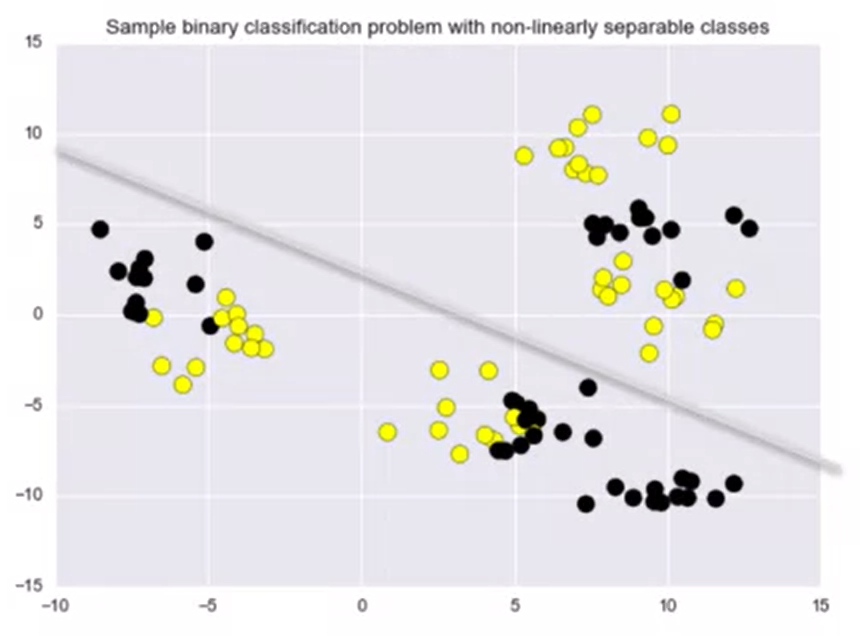## Kernelized Support Vector Machines

Details of Kernelized svm will be released later.

sklearn.svm.SVC — scikit-learn 0.24.2 documentation

### Application

Classification

from sklearn.svm import SVC

X_train, X_test, y_train, y_test = train_test_split(X_D2, y_D2, random_state = 0)

# The default SVC kernel is radial basis function (RBF)
plot_class_regions_for_classifier(SVC().fit(X_train, y_train),
X_train, y_train, None, None,
'Support Vector Classifier: RBF kernel')

# Compare decision boundries with polynomial kernel, degree = 3
plot_class_regions_for_classifier(SVC(kernel = 'poly', degree = 3)
.fit(X_train, y_train), X_train,
y_train, None, None,
'Support Vector Classifier: Polynomial kernel, degree = 3')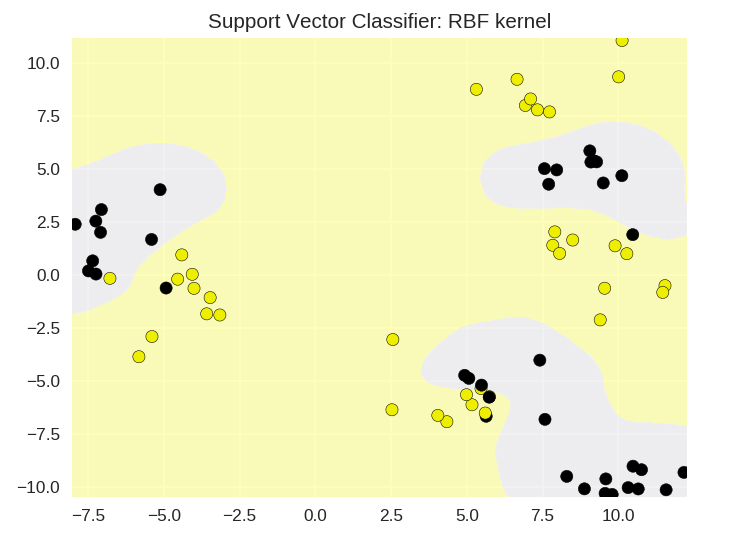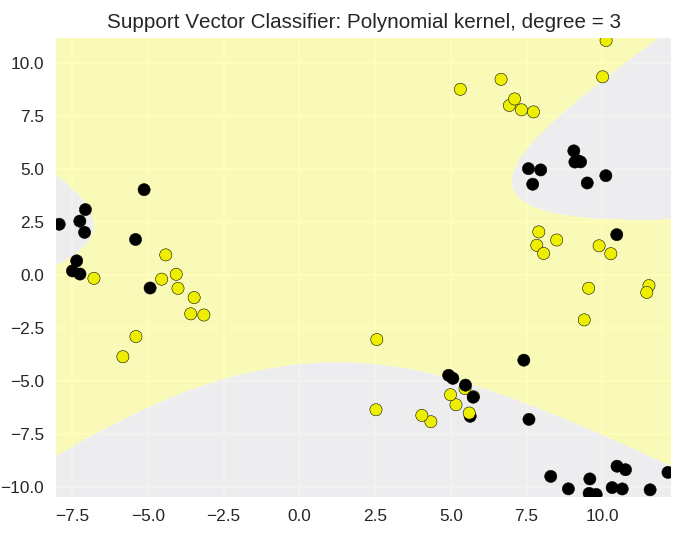### How gamma and C affect result

from sklearn.svm import SVC

from sklearn.model_selection import train_test_split

X_train, X_test, y_train, y_test = train_test_split(X_D2, y_D2, random_state = 0)
fig, subaxes = plt.subplots(3, 4, figsize=(15, 10), dpi=50)

for this_gamma, this_axis in zip([0.01, 1, 5], subaxes):

for this_C, subplot in zip([0.1, 1, 15, 250], this_axis):
title = 'gamma = {:.2f}, C = {:.2f}'.format(this_gamma, this_C)
clf = SVC(kernel = 'rbf', gamma = this_gamma,
C = this_C).fit(X_train, y_train)
plot_class_regions_for_classifier_subplot(clf, X_train, y_train,
X_test, y_test, title,
subplot)Gamma(γ)：Controls how far the influence of a single trending example reaches

larger gamma ▶️ smaller similarity radius ▶️ sharpen the decision boundaries

C Parameter: Controls the tradeoff between satisfying the maximum margin criterion to find the simple decision boundary and avoiding the classification errors.

# Decision Tree

sklearn.tree.DecisionTreeClassifier — scikit-learn 0.24.2 documentation

## Application

max_depth: control maximum depth(number of split points). Most common way to reduce tree complexity and overfitting.

min_samples_leaf: threshold for the minimum of data instances a leaf can have to avoid further splitting.

max_leaf_nodes: limit total number of leaves in the tree.

Tips: adjusting only one of these(e.g. max_depth) is enough to reduce overfitting.

from sklearn.datasets import load_iris
from sklearn.tree import DecisionTreeClassifier
from sklearn.model_selection import train_test_split

X_train, X_test, y_train, y_test = train_test_split(iris.data, iris.target, random_state = 3)
clf = DecisionTreeClassifier().fit(X_train, y_train)

print('Accuracy of Decision Tree classifier on training set: {:.2f}'
.format(clf.score(X_train, y_train)))
print('Accuracy of Decision Tree classifier on test set: {:.2f}'
.format(clf.score(X_test, y_test)))


Accuracy of Decision Tree classifier on training set: 1.00
Accuracy of Decision Tree classifier on test set: 0.95

Setting max decision tree depth to help avoid overfitting

clf2 = DecisionTreeClassifier(max_depth = 3).fit(X_train, y_train)

print('Accuracy of Decision Tree classifier on training set: {:.2f}'
.format(clf2.score(X_train, y_train)))
print('Accuracy of Decision Tree classifier on test set: {:.2f}'
.format(clf2.score(X_test, y_test)))


Accuracy of Decision Tree classifier on training set: 0.98
Accuracy of Decision Tree classifier on test set: 0.97

Visualize Decision Tree

plot_decision_tree(clf, iris.feature_names, iris.target_names)


: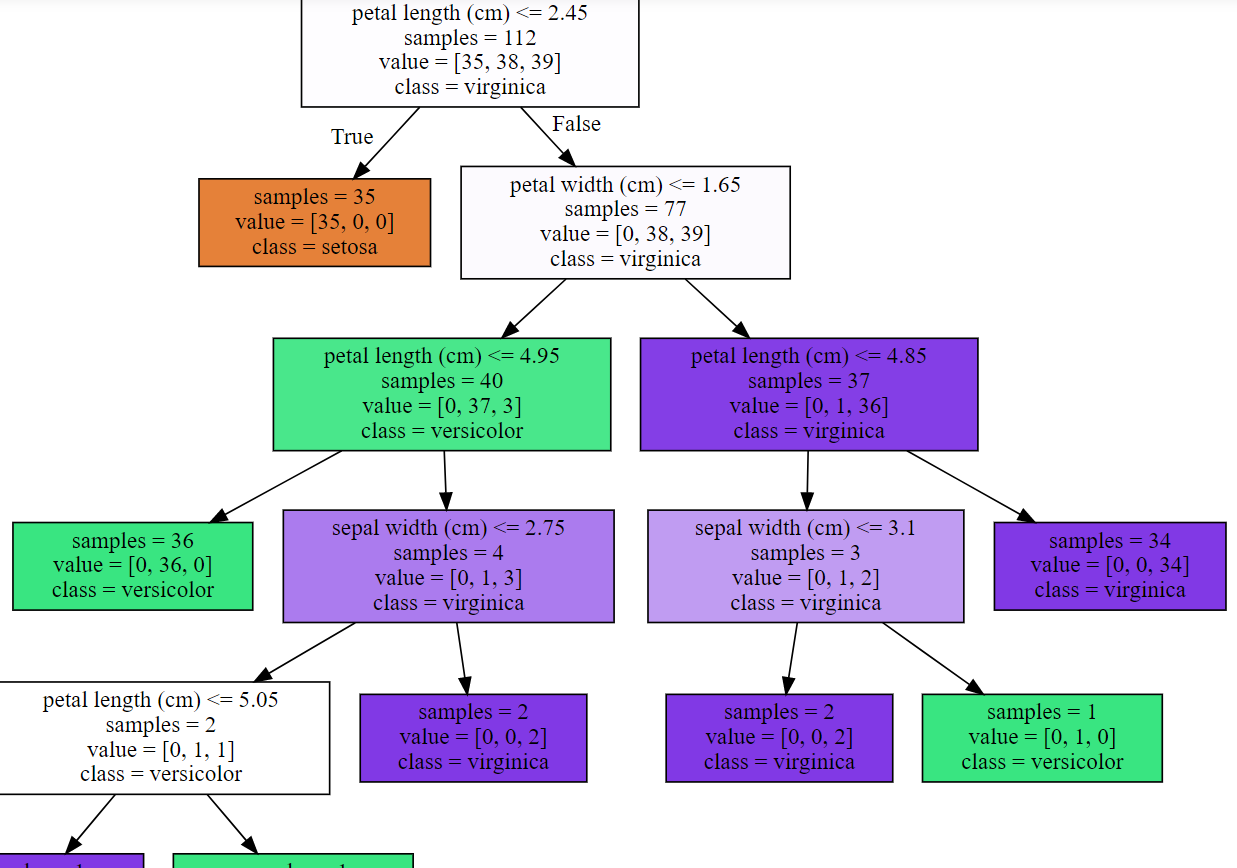## Pros and Cons

1 Breiman, Friedman, “Classification and regression trees”, 1984.
• 814
• 0

### YOU MIGHT ALSO LIKE

0 0 vote
Article Rating
Subscribe0 评论
Inline Feedbacks